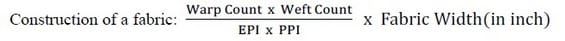# Warping Calculation | Calculation for Direct Warping and Sectional Warping

Last Updated on 17/02/2022

Warping:
In warping process, the yarns are transferred from a number of supply packages (cones) to the warp beam in the form of a parallel sheet. The main objective of warping is to get the required number of ends as per requirement. It is a pre-process of sizing. The warping consists of collecting predetermined number of ends from a set of wound package and transferring them in a sheet form to a weavers beam. The parallel winding of warp ends small package (bobbin) to a common package (warp beam) is called warping.

Or,

The operation of winding warp yarns to a beam usually in preparation for sizing, weaving or warp knitting is called warping.

I have published lots of article on warping. Now I will discuss on warping calculation both direct warping and sectional warping.

Warping Calculation:

Production = Surface speed of drum × π × Dia. of drum × Creel Capacity

Warping calculation related to warp beam preparation is done by the following process.Total no of ends = EPI x Fabric Width
Creel capacity = Total number of ends/number of warp beam required
No. of warp beam = Total ends/creel capacity
Ends per beam = Total no of ends/no. of warp beam

A Typical Calculation for Direct Warping:Total no. of ends = EPI x fabric width
= 100 x 116
= 11600

No. of warp beam = Total no of ends /creel capacity
= 11600/580 (Creel Capacity is available up to 656)
= 20

The no. of cone available in a packet is 24 & total weight of the packet is 100 lbs.

Now 1 cone weight = 100/24
= 4.16 lbs.
= 4.16/0.04 kg
= 1.88 kg

Now 1 cone length = Warp Count x Cone wt. in lbs x 840
= 40 x 4.16 x 840
= 139776 yds
= 139776/1.0936 m
= 127812.73 m

Length Variation = 127812.73 m x 5% (If length variation is 5%)
= 6390.64 m

So, yarn length in 1 cone = Calculated length – length variation
=127812.73 m – 6390.64 m
= 121422.90 m

Length of yarn per warp beam = Total length in 1 cone/no of warp beam
= 121422.90/20
= 6071 m

Now this length will be fixed in warping machine & each time the warp sheet will be cut off after reaching the fixed length wound on the beam. In this way warp beams will be wound continuously. Then it goes to sizing section.

Production = Surface speed of drum × π × Dia. of drum × Creel Capacity
= 20.65 × 3.1416 × 30” × 580
= 1128808.3 inch/ min
= (1128808.3/39.37) × 60 m/hr
= 1720307.3 m/hr

A Typical Calculation for Sectional Warping:

Available Creel capacity = 560
Total no. of ends per pattern = 42

So, no. of pattern per section = Creel Capacity/Total ends in pattern
= 560 / 42
= 13.33
≈12 pattern (As required)

Creel to be used = Total pattern x Total ends in pattern
= 12 x 42
= 504

Total section = Total Ends / Creel used
= 7000 / 504
= 14 sections

Production = Surface speed of drum × π × Dia. of drum × Creel Capacity
= 20.65 × 3.1416 × 30” × 504
= 980895.48 inch/ min
= (980895.48/39.37) × 60 m/hr
= 1494887.7 m/hr

You may also like: